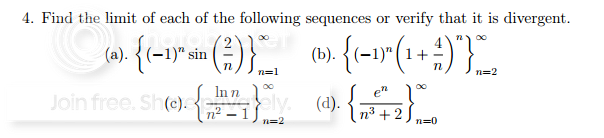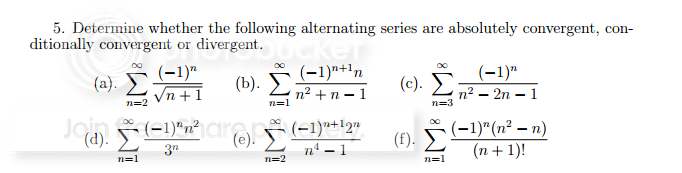# Convergence and Divergence - Calculus 2

#### balloonhf

##### New member
Alright, I have my final for Calc 2 on Monday. I am only stuck on two sections of problems because I am terrible at convergence and divergence/alternating series.I have questions for each one really. They are as follows:
4)
a. Can I simply factor out the alternating series and apply a test since the alternating portion is constant?
b. Similar to my question from a.
c. Not sure of what test to apply for convergence tests. I have tried both the nth root test and the ratio test and neither work.
d. Similar to my question from c.

5)
a) Again can I factor out the alternating series and say that it is similar to 1/(n)^(1/2) and 1/2<1 so it diverges.
b) and c) - I have literally no idea.
d)Same as a in that I want to know if i can factor out the constant alternating section
e) and f) - No idea

I have a general idea on most problems. Most of my questions are on the same thing that I need confirmed. Also I know the tests for Absolute and Conditionally convergent.

Any sort of help or confirmation on my assumptions is greatly appreciated.

#### ZaidAlyafey

##### Well-known member
MHB Math Helper
a. Can I simply factor out the alternating series and apply a test since the alternating portion is constant?
b. Similar to my question from a.
See this .

c. Not sure of what test to apply for convergence tests. I have tried both the nth root test and the ratio test and neither work.
Here you can use the comparison test .

d. Similar to my question from c.
Try the divergence test .

5)
a) Again can I factor out the alternating series and say that it is similar to 1/(n)^(1/2) and 1/2<1 so it diverges.
b) and c) - I have literally no idea.
d)Same as a in that I want to know if i can factor out the constant alternating section
If the non-alternating part is positive and decreasing , then it is sufficient to test whether it goes to 0 as n goes to infinity.

e) and f) - No idea
Try the divergence test on e , and f can be simplified a little ,see how ?

#### balloonhf

##### New member
For a. Can I just state that sin is bounded by -1 to 1 and therefore does not exist?

I went to the link you provided. I set bn=sin(1/n) and the limit does not equal 0, and therefore does not converge?

Also the same with b. Since the lim of bn which would be (1 +4/n)^n would also = 1 and not converge.

edit: reading further and discovered the next step.

Alright so by the divergence test as well I can confirm that both A and B diverge?

Last edited:

#### balloonhf

##### New member
Here you can use the comparison test .
For C I tried setting bn=1/(n^(2) -1) and took the limit to get that it goes to 0. Since bn is not greater than 0 an also converges.

Yes?
If so then I did the same thing for D and got the same answer.

Last edited:

#### ZaidAlyafey

##### Well-known member
MHB Math Helper
For a. Can I just state that sin is bounded by -1 to 1 and therefore does not exist?

I went to the link you provided. I set bn=sin(1/n) and the limit does not equal 0, and therefore does not converge?

Also the same with b. Since the lim of bn which would be (1 +4/n)^n would also = 1 and not converge.

edit: reading further and discovered the next step.

Alright so by the divergence test as well I can confirm that both A and B diverge?
Sorry, the first question is regarding sequences . So you test sin(1/n) as n goes to infinity , what is the limit ?

#### ZaidAlyafey

##### Well-known member
MHB Math Helper
For C I tried setting bn=1/(n^(2) -1) and took the limit to get that it goes to 0. Since bn is not greater than 0 an also converges.

Yes?
If so then I did the same thing for D and got the same answer.
$$\displaystyle \frac{\ln (n)}{n^2-1}< \frac{n}{n^2-1}$$

Sine the later converges , by comparison test the previous converges .